Open in App
Not now

# How to Impute Missing Values in R?

• Last Updated : 04 Jan, 2022

In this article, we will discuss how to impute missing values in R programming language.

In most datasets, there might be missing values either because it wasn’t entered or due to some error. Replacing these missing values with another value is known as Data Imputation. There are several ways of imputation. Common ones include replacing with average, minimum, or maximum value in that column/feature. Different datasets and features will require one type of imputation method. For example, considering a dataset of sales performance of a company, if the feature loss has missing values then it would be more logical to replace a minimum value.

### Dataset in use: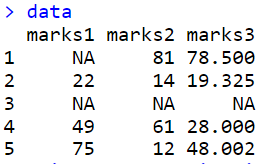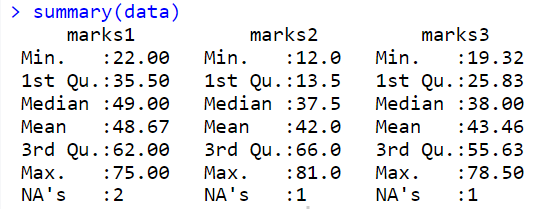## Impute One Column

### Method 1: Imputing manually with Mean value

Let’s impute the missing values of one column of data, i.e marks1 with the mean value of this entire column.

Syntax  :

mean(x, trim = 0, na.rm = FALSE, …)

Parameter:

• x – any object
• trim – observations to be trimmed from each end of x before the mean is computed
• na.rm – FALSE to remove NA values

Example: Imputing missing values

## R

 `# create a adataframe ` `data <- ``data.frame``(marks1 = ``c``(``NA``, 22, ``NA``, 49, 75), ` `                   ``marks2 = ``c``(81, 14, ``NA``, 61, 12), ` `                   ``marks3 = ``c``(78.5, 19.325, ``NA``, 28, 48.002)) ` ` `  `# impute manually ` `data\$marks1[``is.na``(data\$marks1)] <- ``mean``(data\$marks1, na.rm = T)   ` ` `  `data `

Output: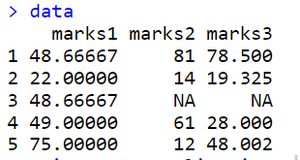### Method 2: Using Hmisc Library and imputing with Median value

Using the function impute( ) inside Hmisc library let’s impute the column marks2 of data with the median value of this entire column.

Example: Impute missing values

## R

 `# install and load the required packages ` ` `  `install.packages``(``"Hmisc"``) ` `library``(Hmisc) ` ` `  `# create a adataframe ` `data <- ``data.frame``(marks1 = ``c``(``NA``, 22, ``NA``, 49, 75), ` `                   ``marks2 = ``c``(81, 14, ``NA``, 61, 12), ` `                   ``marks3 = ``c``(78.5, 19.325, ``NA``, 28, ` `                              ``48.002)) ` ` `  `# fill missing values of marks2 with median ` `impute``(data\$marks2, median)`

Output: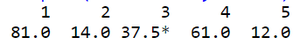imputing with Median value

### Method 3: Impute with a specific Constant value

Using the function impute( ) inside Hmisc library let’s impute the column marks2 of data with a constant value.

Example: Impute missing values

## R

 `# install and load the required packages ` `install.packages``(``"Hmisc"``) ` `library``(Hmisc) ` ` `  `# create a adataframe ` `data <- ``data.frame``(marks1 = ``c``(``NA``, 22, ``NA``, 49, 75), ` `                   ``marks2 = ``c``(81, 14, ``NA``, 61, 12), ` `                   ``marks3 = ``c``(78.5, 19.325, ``NA``, 28,  ` `                              ``48.002)) ` ` `  `# impute with a specific number ` `# replace NA with 2000 ` `impute``(data\$marks3, 2000)   `

Output: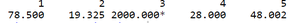Impute with a specific Constant value

## Impute the entire dataset:

This can be done by imputing Median value of each column with NA using apply( ) function.

Syntax:

apply(X, MARGIN, FUN, …)

Parameter:

• X – an array, including a matrix
• MARGIN – a vector
• FUN – the function to be applied

Example: Impute the entire dataset

## R

 `# create a adataframe ` `data <- ``data.frame``(marks1 = ``c``(``NA``, 22, ``NA``, 49, 75), ` `                   ``marks2 = ``c``(81, 14, ``NA``, 61, 12), ` `                   ``marks3 = ``c``(78.5, 19.325, ``NA``, 28,  ` `                              ``48.002)) ` ` `  `# getting median of each column using apply()  ` `all_column_median <- ``apply``(data, 2, median, na.rm=``TRUE``) ` ` `  `# imputing median value with NA  ` `for``(i ``in` `colnames``(data)) ` `  ``data[,i][``is.na``(data[,i])] <- all_column_median[i] ` ` `  `data`

Output: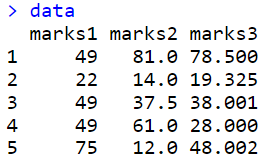My Personal Notes arrow_drop_up
Related Articles